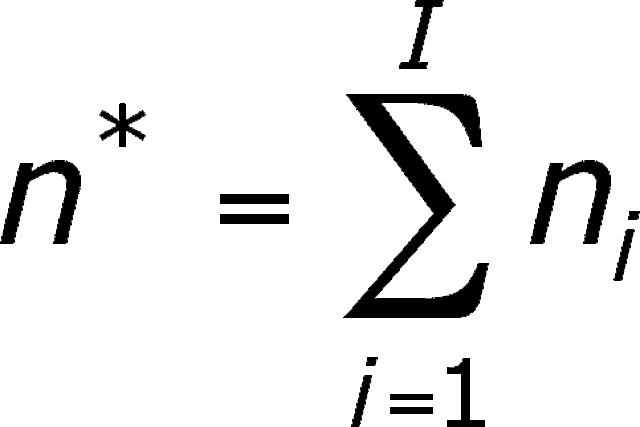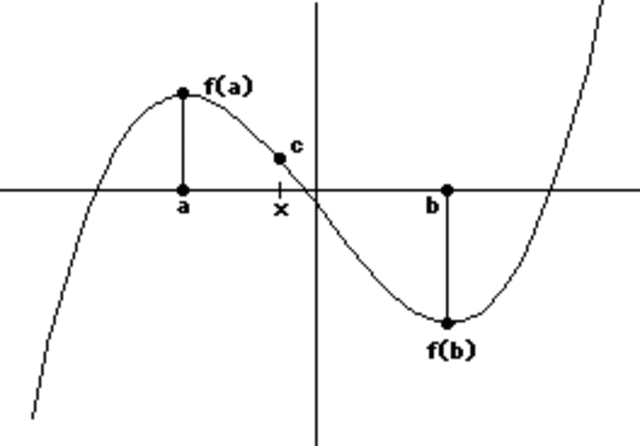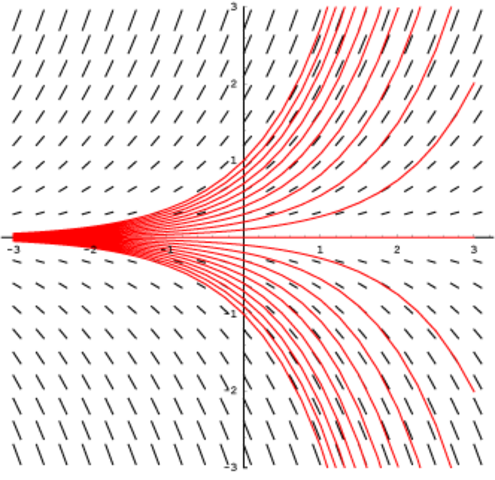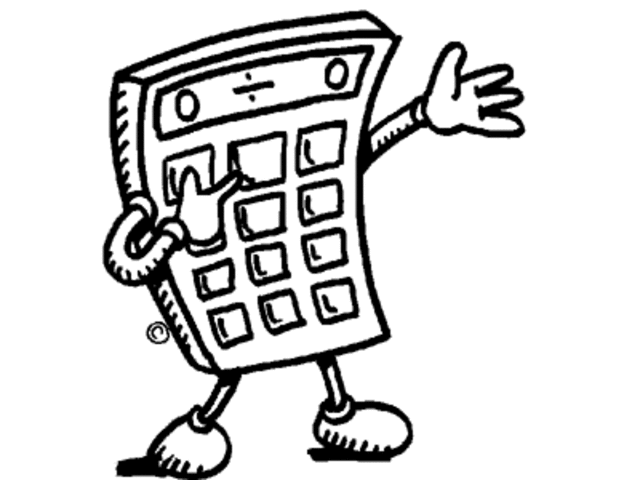# Calculus Journal Page

Timeline created by jaysimms123
• # Sequences and SeriesSequence is a set of numbers
Series is a sum of a sequence
REMEMBER to pay attention to the wording and were it starts from (n=1 or 0)
monotonic always increasing or decreasing.
• # Intermediant Value therom and reviewThis was a helpful day because this always shows up on the test.
If there is two points a and b there must be a point c in between them like shown in the picture
• # Slope Feilds and eulers method and logisticsslope feilds are just a depiction of the derivitive of a funtion. eulers method is to solve differential equations at a given point. logistics can model exponential growth and decay. There can also be a maximum on this which is l/2
• # FIrst AP problemsneed to becareful of time. remember i can use a calculator on the first 3 problemss.
• # More AP problemsReview the rate of change problems. on this set we had a harder time so we remembered to write down equations and steps to get more points. a useful trick
• # Final AP problemsfound these were the easiest of all the problmes. bradley and i got through them quickly trying to get the maximum of points.